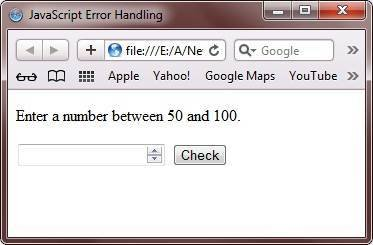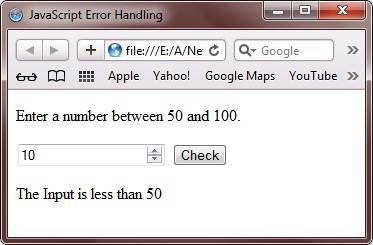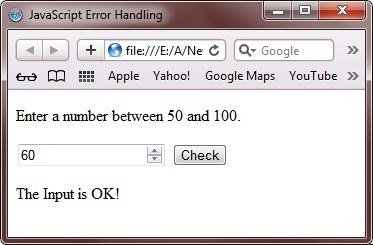# JavaScript Error Handling

There are many errors which can arise on executing your JavaScript code. Therefore, you must have to be aware, how to handle errors.

Error handling in JavaScript can be carried out with JavaScript try-catch statement.

## JavaScript Error Handling Example

Here is an example on error handling in JavaScript:

```<!DOCTYPE html>
<html>
<head>
<title>JavaScript Error Handling</title>
</head>
<body>

<p>Enter a number between 50 and 100.</p>

<input id="demoinp" type="number" min="50" max="100" step="1">
<button type="button" onclick="myerrhandle()">Check</button>
<p id="errormessage"></p>

<script>
function myerrhandle()
{
var message, x;
message = document.getElementById("errormessage");
message.innerHTML = "";
x = document.getElementById("demoinp").value;
try
{
if(x == "")
throw "empty";
if(isNaN(x))
throw "not a number";
x == Number(x);
if(x>50 && x<100)
throw "OK!";
if(x < 50)
throw "less than 50";
if(x > 100)
throw "greater than 100";
}
catch(err)
{
message.innerHTML = "The Input is " + err;
}
}
</script>

</body>
</html>```

Here is the sample output produced by the above JavaScript error handling example code. This is the initial output:Now, enter a number and press on the Check button to produce the output as shown in the following snapshot:Here is another sample run of the above JavaScript error handling program:Below is one more sample run:Here is the live demo output of the above JavaScript error handling example program. Enter any number and press on the Check button to check the output:

Enter a number between 50 and 100.

Tools
Calculator

Quick Links
Signup - Login - Give Online Test